# GeetCode Hub

Given two binary trees `original` and `cloned` and given a reference to a node `target` in the original tree.

The `cloned` tree is a copy of the `original` tree.

Return a reference to the same node in the `cloned` tree.

Note that you are not allowed to change any of the two trees or the `target` node and the answer must be a reference to a node in the `cloned` tree.

Follow up: Solve the problem if repeated values on the tree are allowed.

Example 1: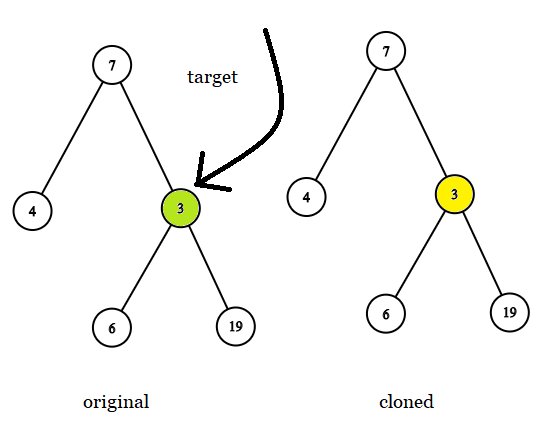```Input: tree = [7,4,3,null,null,6,19], target = 3
Output: 3
Explanation: In all examples the original and cloned trees are shown. The target node is a green node from the original tree. The answer is the yellow node from the cloned tree.
```

Example 2: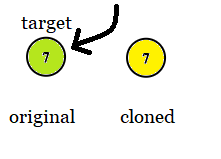```Input: tree = , target =  7
Output: 7
```

Example 3: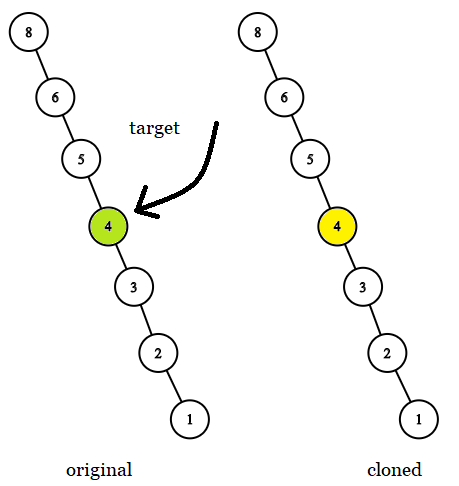```Input: tree = [8,null,6,null,5,null,4,null,3,null,2,null,1], target = 4
Output: 4
```

Example 4: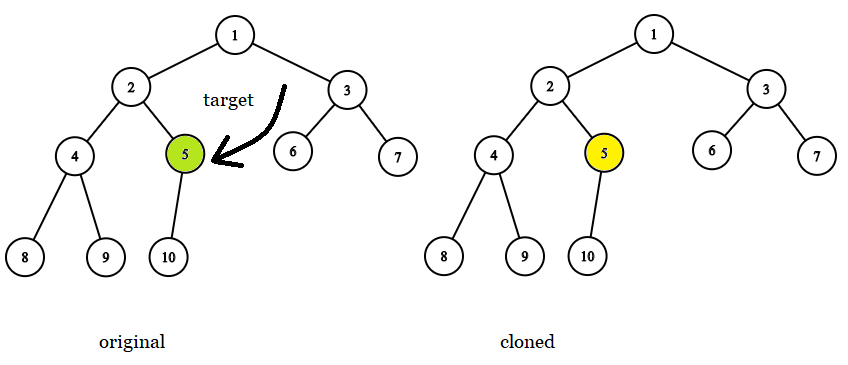```Input: tree = [1,2,3,4,5,6,7,8,9,10], target = 5
Output: 5
```

Example 5: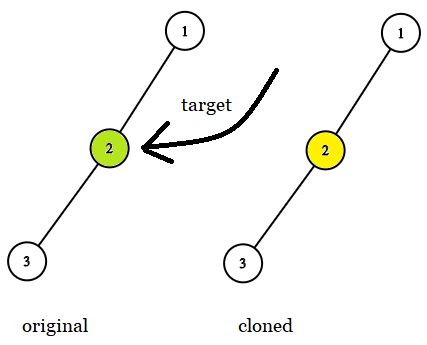```Input: tree = [1,2,null,3], target = 2
Output: 2
```

Constraints:

• The number of nodes in the `tree` is in the range `[1, 10^4]`.
• The values of the nodes of the `tree` are unique.
• `target` node is a node from the `original` tree and is not `null`.

/** * Definition for a binary tree node. * public class TreeNode { * int val; * TreeNode left; * TreeNode right; * TreeNode(int x) { val = x; } * } */ class Solution { public final TreeNode getTargetCopy(final TreeNode original, final TreeNode cloned, final TreeNode target) { } }